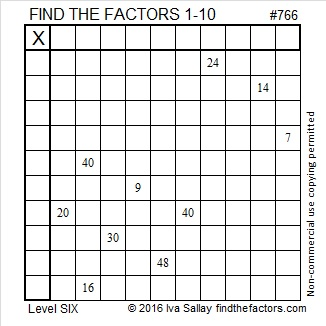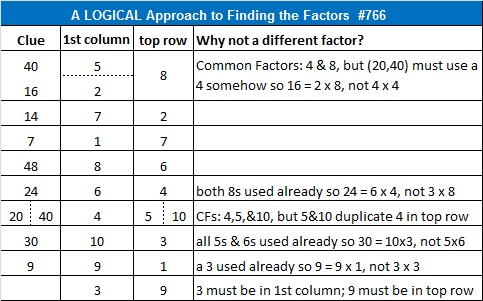# 766 and Level 6

• 766 is a composite number.
• Prime factorization: 766 = 2 x 383
• The exponents in the prime factorization are 1 and 1. Adding one to each and multiplying we get (1 + 1)(1 + 1) = 2 x 2 = 4. Therefore 766 has exactly 4 factors.
• Factors of 766: 1, 2, 383, 766
• Factor pairs: 766 = 1 x 766 or 2 x 383
• 766 has no square factors that allow its square root to be simplified. √766 ≈ 27.6767.Here’s a level 6 puzzle for you to try:Print the puzzles or type the solution on this excel file: 10 Factors 2016-02-04

—————————————

Here are a few more reasons to be interested in the number 766:

766 is the sum of the twelve prime numbers from 41 to 89.

766 is palindrome 23332 in BASE 4; note that 2(256) + 3(64) + 3(16) + 3(4) + 2(1) = 766.

766 is the sum of three squares six different ways:

• 27² + 6² + 1² = 766
• 26² + 9² + 3² = 766
• 21² + 18² + 1² = 766
• 21² + 17² + 6² = 766
• 21² + 15² + 10² = 766
• 19² + 18² + 9² = 766

766 is also the 18th centered pentagonal number because (5⋅17² + 5⋅17 + 2)/2 = 766.

—————————————

This table shows a way to solve puzzle #766 using logic: